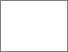# Predicting toxic gas concentrations resulting from enclosure fires using the local equivalence ratio concept linked to fire field models

Wang, Zhaozhi (2007) Predicting toxic gas concentrations resulting from enclosure fires using the local equivalence ratio concept linked to fire field models. PhD thesis, University of Greenwich.Preview
PDF (Pages containing signatures redacted)
Zhaozhi Wang 2007 - Redacted.pdf - Published Version

## Abstract

Fire field modelling is based on the techniques of computational fluid dynamics (CFD), which provide detailed variable solutions throughout the computational domain quickly, repeatedly and cheaply compared with fire experiments. A main component of fire effluent are toxic gases and one of the main toxic gases responsible for a significant number of fire fatalities is Carbon Monoxide (CO). Current engineering fire field models either ignore the prediction of toxic gas generation or require the use of detailed chemistry to predict toxic gas generation. These detailed chemistry models require considerable data which is not generally available for most common building materials. The main objective of the study presented in this thesis is to develop practical and reasonable engineering fire field models to simulate the production and movement of toxic gases in enclosure fires. The developed toxicity models should be capable of working within the framework of the current popular combustion models in fire safety engineering. In addition, the developed models should not rely too heavily on hard to obtain experimental data.

The central idea behind the newly developed toxicity model is the use of the Local Equivalence Ratio (LER). The species yields as functions of the Global Equivalence Ratio (GER) and temperature are input parameters of this model. Correlations for most building materials are available from small-scale fire experiments. Similar approaches to this method are also developed using the CO/CO2 and H2/H2O mole ratios. The LER methodology is further refined by an approach which divides the computational domain for the calculation of toxic gases into two parts, a control region in which the toxic gases are dependent on the LER and temperature, and a transport region in which the toxic gas concentrations are dependent on the mixing of hot gases with fresh air.

The toxicity model is then extended to two-fuel cases. In the two-fuel model, the LER is a function of the two mixture fractions, which are used to represent the mixture of the two different fuels, oxygen and combustion products. This model is useful in simulating residential fires, in which wood lining of sidewalls or ceilings is the second fuel.

Finally, the transportation of HCI within fire compartments is considered. A mathematical model is developed to simulate the exchange of HCI between gas boundary and wall surfaces and the reaction of HCI with walls.

All the toxicity models developed in this study can be integrated into the practical volumetric heat source approach and the Eddy Break-up (EBU) combustion model typically used in practical engineering analysis.

Item Type: Thesis (PhD) uk.bl.ethos.437713 mathematical modelling, fire safety, fire simulation, H Social Sciences > HD Industries. Land use. LaborQ Science > QA MathematicsT Technology > TH Building construction School of Computing & Mathematical SciencesSchool of Computing & Mathematical Sciences > Centre for Numerical Modelling & Process Analysis > Fire Safety Engineering GroupSchool of Computing & Mathematical Sciences > Department of Mathematical Sciences Organisation 17 Jan 2019 15:02 http://gala.gre.ac.uk/id/eprint/6338View Item